# 5.3 Solve systems of equations by elimination  (Page 2/6)

 Page 2 / 6

Once we get an equation with just one variable, we solve it. Then we substitute that value into one of the original equations to solve for the remaining variable. And, as always, we check our answer to make sure it is a solution to both of the original equations.

Now we’ll see how to use elimination to solve the same system of equations we solved by graphing and by substitution.

## How to solve a system of equations by elimination

Solve the system by elimination. $\left\{\begin{array}{c}2x+y=7\hfill \\ x-2y=6\hfill \end{array}$

## Solution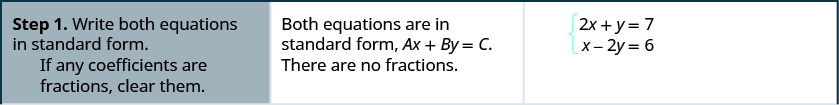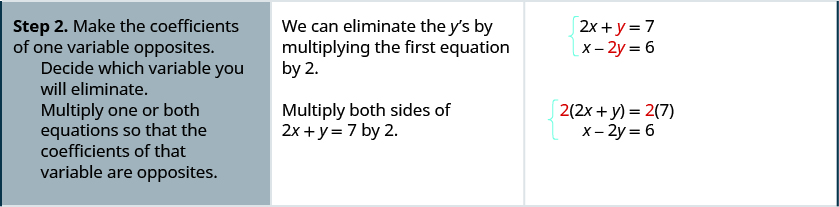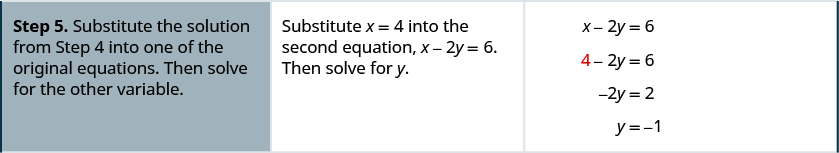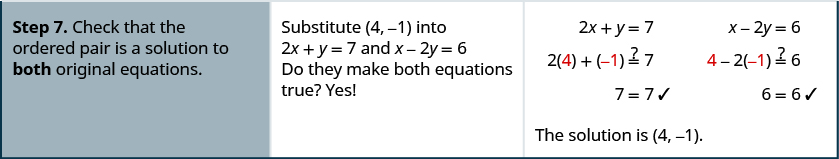Solve the system by elimination. $\left\{\begin{array}{c}3x+y=5\hfill \\ 2x-3y=7\hfill \end{array}$

$\left(2,-1\right)$

Solve the system by elimination. $\left\{\begin{array}{c}4x+y=-5\hfill \\ -2x-2y=-2\hfill \end{array}$

$\left(-2,3\right)$

The steps are listed below for easy reference.

## How to solve a system of equations by elimination.

1. Write both equations in standard form. If any coefficients are fractions, clear them.
2. Make the coefficients of one variable opposites.
• Decide which variable you will eliminate.
• Multiply one or both equations so that the coefficients of that variable are opposites.
3. Add the equations resulting from Step 2 to eliminate one variable.
4. Solve for the remaining variable.
5. Substitute the solution from Step 4 into one of the original equations. Then solve for the other variable.
6. Write the solution as an ordered pair.
7. Check that the ordered pair is a solution to both original equations.

First we’ll do an example where we can eliminate one variable right away.

Solve the system by elimination. $\left\{\begin{array}{c}x+y=10\hfill \\ x-y=12\hfill \end{array}$

## Solution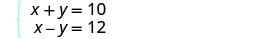Both equations are in standard form. The coefficients of y are already opposites. Add the two equations to eliminate y . The resulting equation has only 1 variable, x .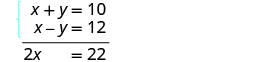Solve for x , the remaining variable. Substitute x = 11 into one of the original equations.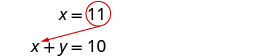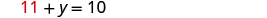Solve for the other variable, y .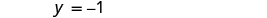Write the solution as an ordered pair. The ordered pair is (11, −1). Check that the ordered pair is a solution to both original equations. $\begin{array}{cccc}\begin{array}{ccc}\hfill x+y& =\hfill & 10\hfill \\ \hfill 11+\left(-1\right)& \stackrel{?}{=}\hfill & 10\hfill \\ \hfill 10& =\hfill & 10\phantom{\rule{0.2em}{0ex}}✓\hfill \end{array}& & & \begin{array}{ccc}\hfill x-y& =\hfill & 12\hfill \\ \hfill 11-\left(-1\right)& \stackrel{?}{=}\hfill & 12\hfill \\ \hfill 12& =\hfill & 12\phantom{\rule{0.2em}{0ex}}✓\hfill \end{array}\end{array}$ The solution is (11, −1).

Solve the system by elimination. $\left\{\begin{array}{c}2x+y=5\hfill \\ x-y=4\hfill \end{array}$

$\left(3,-1\right)$

Solve the system by elimination. $\left\{\begin{array}{c}x+y=3\hfill \\ -2x-y=-1\hfill \end{array}$

$\left(-2,5\right)$

In [link] , we will be able to make the coefficients of one variable opposites by multiplying one equation by a constant.

Solve the system by elimination. $\left\{\begin{array}{c}3x-2y=-2\hfill \\ 5x-6y=10\hfill \end{array}$

## Solution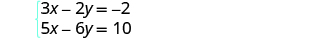Both equations are in standard form. None of the coefficients are opposites. We can make the coefficients of y opposites by multiplying the first equation by −3.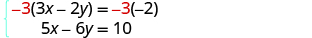Simplify.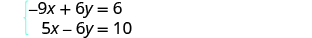Add the two equations to eliminate y .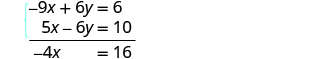Solve for the remaining variable, x . Substitute x = −4 into one of the original equations.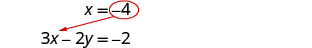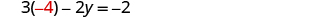Solve for y .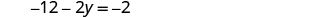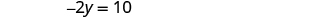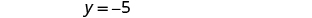Write the solution as an ordered pair. The ordered pair is (−4, −5). Check that the ordered pair is a solution to both original equations. $\begin{array}{cccc}\begin{array}{ccc}\hfill 3x-2y& =\hfill & -2\hfill \\ \hfill 3\left(-4\right)-2\left(-5\right)& \stackrel{?}{=}\hfill & -2\hfill \\ \hfill -12+10& \stackrel{?}{=}\hfill & -2\hfill \\ \hfill -2y& =\hfill & -2\phantom{\rule{0.2em}{0ex}}✓\hfill \end{array}& & & \begin{array}{ccc}\hfill 5x-6y& =\hfill & 10\hfill \\ \hfill 3\left(-4\right)-6\left(-5\right)& \stackrel{?}{=}\hfill & 10\hfill \\ \hfill -20+30& \stackrel{?}{=}\hfill & 10\hfill \\ \hfill 10& =\hfill & 10\phantom{\rule{0.2em}{0ex}}✓\hfill \end{array}\end{array}$ The solution is (−4, −5).

Solve the system by elimination. $\left\{\begin{array}{c}4x-3y=1\hfill \\ 5x-9y=-4\hfill \end{array}$

$\left(1,1\right)$

Solve the system by elimination. $\left\{\begin{array}{c}3x+2y=2\hfill \\ 6x+5y=8\hfill \end{array}$

$\left(-2,4\right)$

Now we’ll do an example where we need to multiply both equations by constants in order to make the coefficients of one variable opposites.

Solve the system by elimination. $\left\{\begin{array}{c}4x-3y=9\hfill \\ 7x+2y=-6\hfill \end{array}$

## Solution

In this example, we cannot multiply just one equation by any constant to get opposite coefficients. So we will strategically multiply both equations by a constant to get the opposites.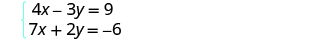Both equations are in standard form. To get opposite coefficients of y , we will multiply the first equation by 2 and the second equation by 3.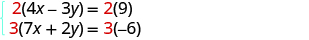Simplify.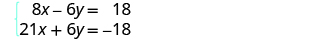Add the two equations to eliminate y .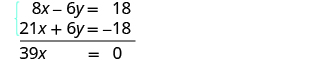Solve for x . Substitute x = 0 into one of the original equations.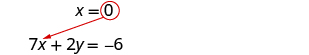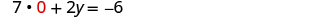Solve for y .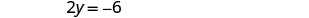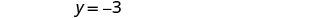Write the solution as an ordered pair. The ordered pair is (0, −3). Check that the ordered pair is a solution to both original equations. $\begin{array}{cccc}\begin{array}{ccc}\hfill 4x-3y& =\hfill & 9\hfill \\ \hfill 4\left(0\right)-3\left(-3\right)& \stackrel{?}{=}\hfill & 9\hfill \\ \hfill 9& =\hfill & 9\phantom{\rule{0.2em}{0ex}}✓\hfill \end{array}& & & \begin{array}{ccc}\hfill 7x+2y& =\hfill & -6\hfill \\ \hfill 7\left(0\right)+2\left(-3\right)& \stackrel{?}{=}\hfill & -6\hfill \\ \hfill -6& =\hfill & -6\phantom{\rule{0.2em}{0ex}}✓\hfill \end{array}\end{array}$ The solution is (0, −3).

What other constants could we have chosen to eliminate one of the variables? Would the solution be the same?

#### Questions & Answers

find the solution to the following functions, check your solutions by substitution. f(x)=x^2-17x+72
Carlos Reply
Aziza is solving this equation-2(1+x)=4x+10
Sechabe Reply
No. 3^32 -1 has exactly two divisors greater than 75 and less than 85 what is their product?
KAJAL Reply
x^2+7x-19=0 has Two solutions A and B give your answer to 3 decimal places
Adedamola Reply
please the answer to the example exercise
Patricia Reply
3. When Jenna spent 10 minutes on the elliptical trainer and then did circuit training for20 minutes, her fitness app says she burned 278 calories. When she spent 20 minutes onthe elliptical trainer and 30 minutes circuit training she burned 473 calories. How manycalories does she burn for each minute on the elliptical trainer? How many calories doesshe burn for each minute of circuit training?
Edwin Reply
.473
Angelita
?
Angelita
John left his house in Irvine at 8:35 am to drive to a meeting in Los Angeles, 45 miles away. He arrived at the meeting at 9:50. At 3:30 pm, he left the meeting and drove home. He arrived home at 5:18.
DaYoungan Reply
p-2/3=5/6 how do I solve it with explanation pls
Adedamola Reply
P=3/2
Vanarith
1/2p2-2/3p=5p/6
James
don't understand answer
Cindy
4.5
Ruth
is y=7/5 a solution of 5y+3=10y-4
Adedamola Reply
yes
James
don't understand answer
Cindy
Lucinda has a pocketful of dimes and quarters with a value of $6.20. The number of dimes is 18 more than 3 times the number of quarters. How many dimes and how many quarters does Lucinda have? Rhonda Reply Find an equation for the line that passes through the point P ( 0 , − 4 ) and has a slope 8/9 . Gabriel Reply is that a negative 4 or positive 4? Felix y = mx + b Felix if negative -4, then -4=8/9(0) + b Felix -4=b Felix if positive 4, then 4=b Felix then plug in y=8/9x - 4 or y=8/9x+4 Felix Macario is making 12 pounds of nut mixture with macadamia nuts and almonds. macadamia nuts cost$9 per pound and almonds cost $5.25 per pound. how many pounds of macadamia nuts and how many pounds of almonds should macario use for the mixture to cost$6.50 per pound to make?
Cherry Reply
Nga and Lauren bought a chest at a flea market for $50. They re-finished it and then added a 350 % mark - up Makaila Reply$1750
Cindy
the sum of two Numbers is 19 and their difference is 15
Abdulai Reply
2, 17
Jose
interesting
saw
4,2
Cindy
Felecia left her home to visit her daughter, driving 45mph. Her husband waited for the dog sitter to arrive and left home 20 minutes, or 13 hour later. He drove 55mph to catch up to Felecia. How long before he reaches her?
Rafi Reply
hola saben como aser un valor de la expresión
NAILEA

### Read also:

#### Get Jobilize Job Search Mobile App in your pocket Now!

Source:  OpenStax, Elementary algebra. OpenStax CNX. Jan 18, 2017 Download for free at http://cnx.org/content/col12116/1.2
Google Play and the Google Play logo are trademarks of Google Inc.

Notification Switch

Would you like to follow the 'Elementary algebra' conversation and receive update notifications?By OpenStaxBy David MartinBy OpenStaxBy OpenStaxBy OpenStaxBy OpenStaxBy OpenStaxBy Abby SharpBy OpenStaxBy Madison Christian